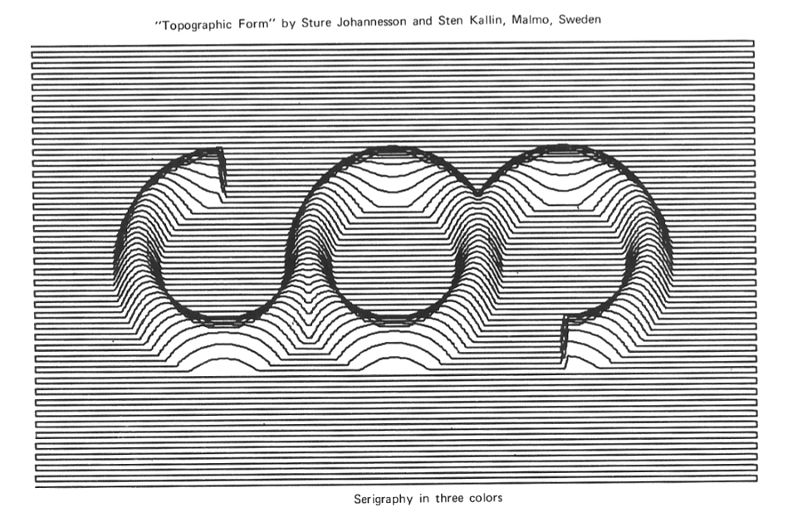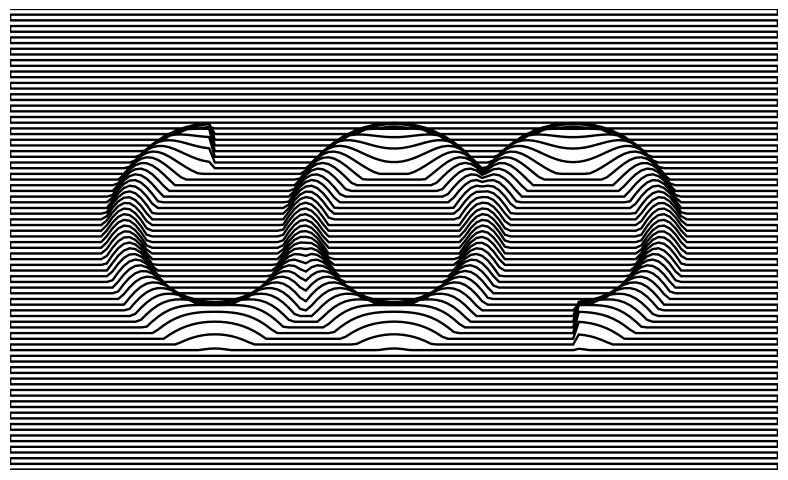# mathr / blog / #

## ReCode Project - Topographic FormPreviously I wrote about the ReCode Project. Today I had a go at another image from the collection, it's not quite pixel perfect but getting pretty close:

import Diagrams.Prelude
import Diagrams.Backend.SVG.CmdLine (defaultMain)

main = defaultMain diagram

diagram = fromVertices zigzag

zigzag = concat $zipWith line ys (cycle [xs, reverse xs]) line y = map (point y) point y x = p2 (x, y + heightMap x y) heightMap x y = maximum [ arcH 36 (\t -> t < 0 || pi/2 < t) x y , arcH 67.5 (\t -> True) x y , arcH 99 (\t -> -pi/2 < t) x y ] arcH x0 f x y | f t && ri < r && r < ro = h0 * cos (pi * (r - r0) / (ro - ri)) | otherwise = 0 where t = atan2 y' x' r = sqrt$ x'^2 + y'^2
x' = x - x0
y' = y - y0

y0 = 40.5
r0 = 15.75
ri = r0 * 0.75
ro = r0 * 1.25
h0 = (ro - ri) / 2
xs = [0 .. 135]
ys = [0 .. 81]